# Chapter 9.2. Problems for Champions – Part II

In this chapter we will review three additional problems that belong to the category "For Champions", i.e. they are more complex than the rest of the problems in this book.

## More Complex Problems on The Studied Material

Before we move on to particular tasks, we must clarify that these can be solved more easily with additional knowledge in programming with JavaScript (functions, arrays, collections, recursion, etc.), but each solution that will be provided now only uses the material covered in this book. The goal is to learn how to construct more complex algorithms based on your knowledge collected up to the present moment.

## Problem: Passion Days

Lina has a real shopping passion. When she has some money, she immediately goes to the closest shopping center (mall) and tries to spend as much as she can on clothes, bags, and shoes. But her favorite thing is winter sales. Our task is to analyze her strange behavior and calculate the purchases that Lina does when she enters the mall, as well as the money she has left when the shopping is over. All prices and money are in BGN (Bulgarian levs, lv).

Our first argument of the function will be the amount that line has before she starts shopping. Our second argument will be a list of commands(strings), which Line will do. After that upon reading "mall.Enter" command, Lina enters the mall and starts shopping until the "mall.Exit" command is given. When Lina starts shopping every next element from the array will be an action that Lina does. Each symbol in the string is a purchase or another action. String commands contain only symbols of the ASCII table. The ASCII code of each sign is related to what Lina must pay for each of the goods. You need to interpret the symbols in the following way:

• If the symbol is a capital letter, Lina gets a 50% discount, which means that you must decrease the money she has by 50% of the numeric representation of the symbol from the ASCII table.
• If the symbol is a small letter, Lina gets a 70% discount, which means that you must decrease the money she has by 30% of the numeric representation of the symbol from the ASCII table.
• If the symbol is "%", Lina makes a purchase that decreases her money in half.
• If the symbol is "*", Lina withdraws money from her debit card and adds 10 lv. to her available funds.
• If the symbol is different from all of the aforementioned, Lina just purchases without discount, and in this case, you should simply subtract the value of the symbol from the ASCII table from her available funds.

If a certain value of her purchases is higher than her current available funds, Lina DOES NOT make the purchase. Lina's funds cannot be less than 0.

The shopping ends when the "mall.Exit" command is given. When this happens, you need to print the number of purchases made and the money that Lina has left.

### Input Data

The input data is two arguments. Our first argument will indicate the amount that Lina has before starting to purchase. Our second argument will be an array of strings that will be processed in the row they are given. After you receive the command "mall.Enter" every next element will be a string containing information about products/actions that Lina wants to do. In the array, we want to perform all the commands until we receive the "mall.Exit" command.

Always only one "mall.Enter" command will be given, as well as only one "mall.Exit" command.

### Output Data

The output data must be printed on the console. When shopping is over, you must print on the console a particular output depending on what purchases have been made.

• If no purchases have been made – "No purchases. Money left: {remaining funds} lv."
• If at least one purchase is made – "{number of purchases} purchases. Money left: {remaining funds} lv."

The funds must be printed with an accuracy of up to 2 symbols after the decimal point.

### Constraints

• Money is a float number within the range: [0 - 7.9 x 1028].
• The number of strings between "mall.Enter" and "mall.Exit" will be within the range: [1-20].
• The number of symbols in each string that represents a command will be within the range: [1-20].
• Allowed execution time: 0.1 seconds.
• Allowed memory: 16 MB.

### Sample Input and Output

Input Output Comment
110
mall.Enter
d
mall.Exit
1 purchases. Money left: 80.00 lv. ‘d’ has an ASCII code of 100. ‘d’ is a small letter, this is why Lina gets a 70% discount. She spends 30% of 100, which is 30 lv. After this purchase, she has: 110 - 30 = 80 lv.
Input Output Input Output
110
['mall.Enter'
'%'
'mall.Exit']
1 purchases. Money left: 55.00 lv. 100
['mall.Enter',
'Ab',
'**',
'mall.Exit']
2 purchases. Money left: 58.10 lv.

### Hints and Guidelines

We will separate the solution of the problem into three main parts:

• Processing of the input.
• Algorithm for solving the problem.
• Formatting the output.

Let's examine each of the parts in detail.

#### Processing The Input Data

The input of our task consists of a few components:

• In the first argument, we have all the money that Lina has for shopping.
• In the second argument, we have an array that is a series of commands.

When we have the money which Lina owns we can step into processing the commands, that we get. But there is a detail that we need to take into consideration. The requirements state the following:

Our second argument will be an array of commands. They will run one after another. When we receive the command "mall.Enter", our next element will be a string containing **information regarding the purchases/actions that Lina wants to perform.

This is where we need to take into consideration the fact that inside our array we should start processing commands, but only after we receive the command "mall.Enter". How can we do that? Using a while or a do-while loop is a good option. Here is an exemplary solution of how to skip all commands before processing the command "mall.Enter":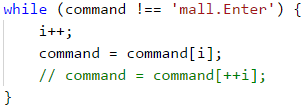We can replace this while with for loop using only the condition and step of the for loop.

Here is the place to say that by calling i++ after the end of the loop is used to pass from the first command for processing because at the end of the loop command[i] points exactly to "mall.Enter", which mustn't be processed as action inside the mall.

#### Algorithm for Solving The Problem

The algorithm for solving the problem is a direct one – we continue reading commands from the console until the command "mall.Exit" is passed. In the meantime, we process each symbol (char) of each one of the commands according to the rules specified in the task requirements, and in parallel, we modify the amount that Lina has and store the number of purchases.

Let's examine the first two problems for our algorithm. The first problem concerns the way we read the commands until we reach the "mall.Exit" command. The solution that we previously saw uses a while-loop. The second problem for the task is to access each symbol of the command passed. Keeping in mind that the input data with the commands is a string type, the easiest way to access each symbol inside the strings is via a foreach loop.

This is how the code will look like: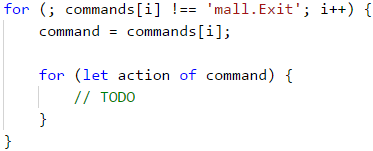The next part of the algorithm is to process the symbols from the commands, according to the following rules in the requirements:

• If the symbol is a capital letter, Lina gets a 50% discount, which means that you must decrease the money she has by 50% of the numeric representation of the symbol from the ASCII table.
• If the symbol is a small letter, Lina gets a 70% discount, which means that you must decrease the money she has by 30% of the numeric representation of the symbol from the ASCII table.
• If the symbol is "%", Lina purchases and that decreases her money in half.
• If the symbol is "*", Lina withdraws money from her debit card and adds 10 lv. to her available funds.
• If the symbol is different from all of the aforementioned, Lina just purchases without discount, and in this case, you should simply subtract the value of the symbol from the ASCII table from her available funds.

Let's examine the problems that we will be facing in the first condition. The first one is how to distinguish if a particular symbol is a capital letter. We can use one of the following ways:

• Keeping in mind the fact that the letters in the alphabet have a particular order, we can use the following condition action >= 'A' && action <= 'Z', to check if our symbol is within the capital letters range.

• We can use the method str.toUpperCase() and compare if the symbol is the same as the one we will receive from str.toUpperCase().

The other problem is how to skip a particular symbol if it is not an operation that requires more money than Lina has. This is doable using the continue construction.

An exemplary condition for the first part of the requirements looks like this: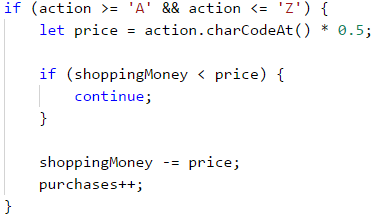Note: the variable “purchases” is of int type, in which we store the number of all purchases.

We believe the reader should not have difficulties implementing all the other conditions because they are very similar to the first one.

#### Formatting The Output

At the end of our task we must print a particular output, depending on the following condition:

• If no purchases have been made – "No purchases. Money left: {remaining funds} lv."
• If at least one purchase is made – "{number of purchases} purchases. Money left: {remaining funds} lv."

The printing operations are trivial, as the only thing we need to take into consideration is that the amount has to be printed with an accuracy of up to 2 symbols after the decimal point.

How can we do that? We will leave the answer to this question to the reader.

### Testing in The Judge System

Test your solution here: https://judge.softuni.org/Contests/Practice/Index/942#0.

## Problem: X Expression

Bonny is an exceptionally powerful witch. As her natural power is not sufficient to successfully fight vampires and werewolves, she has started to master the power of Expressions. An expression is very hard to master because the spell relies on the ability to quickly solve mathematical expressions.

To use an "Expression spell", the witch must know the result of a mathematical expression in advance. An Expression spell consists of a few simple mathematical expressions. Each mathematical expression can contain operators for summing up, subtraction, multiplying, and/or division.

The expression is solved without considering the mathematical rules for calculating numerical expressions. This means that the priority is applied according to the sequence of the operators, and not the type of calculation that they do. The expression can contain brackets, as everything inside the brackets is calculated first. Every expression can contain multiple brackets, but no nested brackets:

• An expression containing (…(…)…) is an invalid one.
• An expression containing (…)…(…) is a valid one.

### Problem

The expression: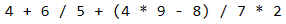is solved in the following way: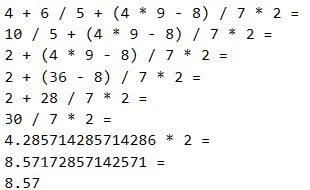Bonny is very pretty, but not as wise, so she will need our help to master the power of Expressions.

### Input Data

The input data consists of a single text line, passed from the console. It contains a mathematical expression for calculation. The line always ends with the "=" symbol. The "=" symbol means the end of the mathematical expression.

The input data is always valid and always in the described format. No need to validate it.

### Output Data

The output data must be printed on the console. The output consists of one line: the result of the calculated mathematical expression.

The result must be rounded up to the second digit after the decimal point.

### Constraints

• The expressions will consist of a maximum of 2500 symbols.
• The numbers of each mathematical expression will be within the range [1 … 9].
• The operators in the mathematical expressions will always be among + (summing up), - (subtraction), / (division) or * (multiplying).
• The result of the mathematical expression will be within the range [-100000.00 … 100000.00].
• Allowed execution time: 0.1 seconds.
• Allowed memory: 16 MB.

### Sample Input and Output

Input Output Input Output
'4+6/5+(4*9-8)/7*2=' 8.57 '3+(6/5)+(2*3/7)*7/2*(9/4+4*1)=' 110.63

### Hints and Guidelines

As usual, we will first read and process the input, after that, we will solve the problem, and finally, we will print the result, formatted as required. In this example, our input will be 1 argument which doesn't need to be processed any further. We can directly go to solving our problem.

#### Algorithm for Solving The Problem

For the tasks of our problem we need to use some variables:

• One variable will store our current result.
• One variable will store the index we are currently on for our loop.
• One variable will store our current index which we process.
• And our final variable will store the current operator from our string.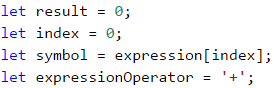Now that we already have our starting variables, we must decide what will be the main structure of our program. By the requirements, we understand that each expression ends with =, i.e. we must read and process symbols until we reach a =. This is followed by an accurately written while loop.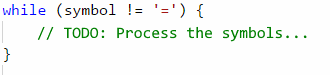Our next step is to process our symbol variable.

The next step is the processing of our symbol variable. We have 3 possible cases for it:

• If the symbol is a start of a sub-expression placed in brackets i.e. the found symbol is a (.
• If the symbol is a digit between 0 and 9. But how can we check this? How can we check if our symbol is a digit? We can use for assistance the ASCII code of the symbol, via which we can use the following formula: [ASCII code of our symbol] – [ASCII code of the symbol 0] = [the digit that represents the symbol]. If the result of this condition is between 0 and 9, then our symbol is a number. ( We can also use directly '0' and '9' symbols or their ASCII codes.)
• If the symbol is an operator, i.e. it is +, -, * or /.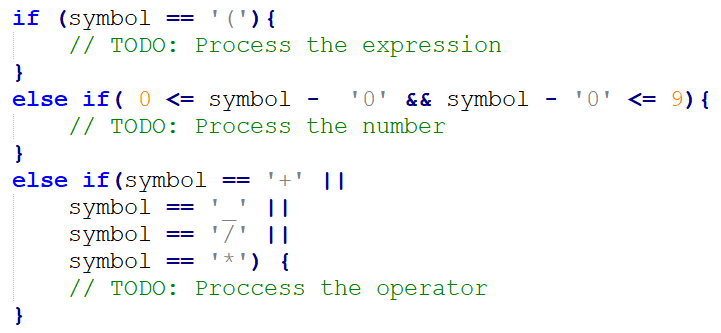Let's examine the actions that we need to undertake in the relevant cases that we defined:

• If our symbol is an operator, then the only thing we need to do is to set a new value for the expressionOperator variable.
• If our symbol is a digit, then we need to change the current result of the expression depending on the current operator, i.e. if expressionOperator is a -, then we must decrease the result by the numerical representation of the current symbol. We can get the numerical representation of the current symbol via the formula that we used upon checking the condition for this case (the [ASCII code of our symbol] – [the ASCII code of the symbol0] = [the digit that represents the symbol])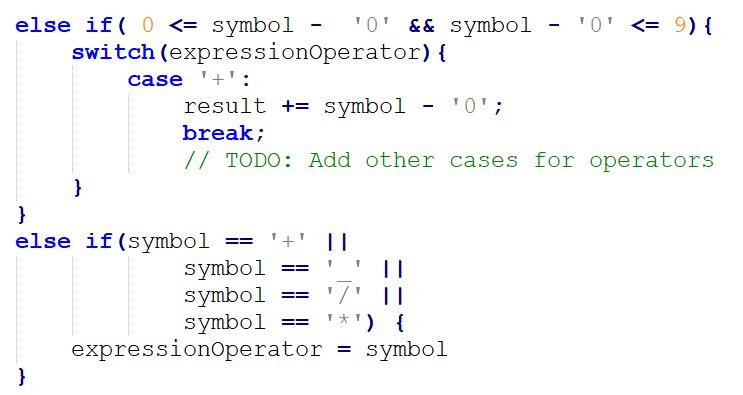• If our symbol is a (, this indicates the beginning of a sub-expression (an expression in brackets). By definition, the sub-expression must be calculated before modifying the result of the whole expression (the actions in brackets are performed first). This means that we will have a local result for the sub-expression and a local operator.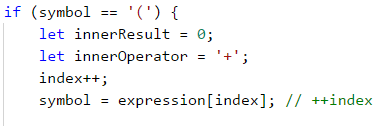After that, to calculate the sub-expression value, we will use the same methods that we used for calculating the main expression – we use a while loop to read symbols (until we reach an ) symbol). Depending on whether the read symbol is a number or an operator, we modify the result of the sub-expression. The implementation of these operations is identical to the above-described implementation for calculating expressions. This is why we believe the reader will be able to easily handle it.

After finishing the result calculation for our sub-expression, we modify the result of the whole expression depending on the value of the expressionOperator.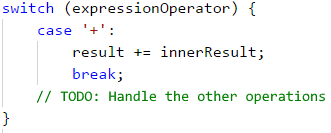#### Formatting The Output

The only output that the program must print on the console is the result of solving the expression with an accuracy of up to two symbols after the decimal point. How can we format the output this way? We will leave the answer to this question to the reader.

### Testing in The Judge System

Test your solution here: https://judge.softuni.org/Contests/Practice/Index/942#1.

## Problem: Bulls and Cows

We all know the game called "Bulls and Cows" (https://en.wikipedia.org/wiki/Bulls_and_cows). Upon having a particular 4-digit secret number and a 4-digit suggested number, the following rules are applied:

• If a digit in the suggested number matches a digit in the secret number and is located at the same position, we have a bull.
• If a digit in the suggested number matches a digit in the secret number but is located at a different position, we have a cow.
Secret number 1 4 8 1 Comment
Suggested number 8 8 1 1 Bulls = 1
Cows = 2
Secret number 2 2 4 1 Comment
Suggested number 9 9 2 4 Bulls = 0
Cows = 2

Upon having a particular secret number and the bulls and cows pertaining to it, our task is to find all possible suggested numbers in ascending order.

If there are no suggested numbers that match the criteria provided from the console, we must print "No".

### Input Data

Our input data consists of 3 arguments:

• The first contains the secret number.
• The second contains the number of bulls.
• The third contains the number of cows.

The input data will always be valid. There is no need to verify them.

### Output Data

The output data must be printed on the console. The output must consist of a single line, holding all suggested numbers, space-separated. If there are no suggested numbers that match the criteria provided from the console, we must print “No”.

### Constraints

• The secret number will always consist of 4 digits in the range [1..9]. [TODO: Digits is good to be unique. If we have a secret number 2132 and suggestion 8762 We have 1 bull and 1 cow for 1 digit.]
• The number of cows and bulls will always be in the range [0..9]. [TODO: Is there a point to have cows and bulls from 0..9? When will we have 5 bulls and 7 cows? The input must be valid.]
• Allowed execution time: 0.15 seconds.
• Allowed memory: 16 MB.

### Sample Input and Output

Input Output
2228
2
1
1222 2122 2212 2232 2242 2252 2262 2272 2281 2283 2284 2285 2286 2287 2289 2292 2322 2422 2522 2622 2722 2821 2823 2824 2825 2826 2827 2829 2922 3222 4222 5222 6222 7222 8221 8223 8224 8225 8226 8227 8229 9222
Input Output
1234
3
0
1134 1214 1224 1231 1232 1233 1235 1236 1237 1238 1239 1244 1254 1264 1274 1284 1294 1334 1434 1534 1634 1734 1834 1934 2234 3234 4234 5234 6234 7234 8234 9234
Input Output
1234
3
1
No

### Hints and Guidelines

Because our input comes directly as an argument of our function we can do the followings steps to complete our task:

• We will generate all possible four-digit combinations (candidates for verification).
• For each generated combination we will calculate how many bulls and how many cows it has according to the secret number. Upon matching the needed bulls and cows, we will print the combination.

#### Algorithm for Solving The Problem

Before starting to write the algorithm for solving our problem, we must declare a flag that indicates whether a solution is found: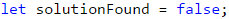If after finishing our algorithm this flag is still false, then we will print No on the console, as specified in the requirements.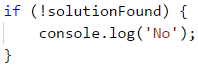Let's start analyzing our problem. What we need to do is analyze all numbers from 1111 to 9999, excluding those that contain zeroes (for example 9011, 3401, etc. are invalid). What is the easiest way to generate all these numbers? We will use nested loops. As we have a 4-digit number, we will have 4 nested loops, as each of them will generate an individual digit in our number for testing.

An alternative solution is to go through all the numbers from 1111 to 999 with a loop and ignore every number with '0' inside it. But this will change the code, which we will do below.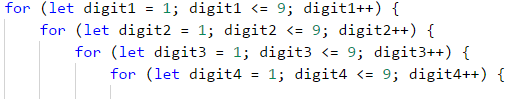Thanks to these loops, we have access to every digit of all numbers that we need to check. Our next step is to separate the secret number into digits. This can be achieved very easily using a combination of integer division and modular division.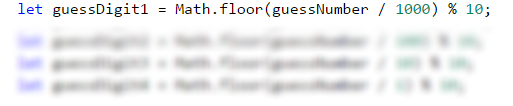How? When we divide we get a decimal number. We can use Math.floor(…) then divide it with a remainder operator(%) by 10, or we can cast the number with parseInt(…). In the example above we remove the fractional part.

Only two last steps remain until we start analyzing how many cows and bulls there are in a particular number. Accordingly, the first one is the declaration of counter variables in the nested loops, to count the cows and bulls for the current number. The second step is to make copies of the digits of the current number that we will analyze, to prevent problems upon working with nested loops, in case we make changes to them.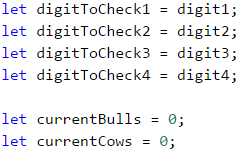We are ready to start analyzing the generated numbers. What logic can we use? The easiest way to check how many cows and bulls there are inside a number is via a sequence of if-else conditions. Yes, this is not the most optimal way, but to stick to what is covered in the current book, we will use this approach.

What conditions do we need?

The condition for the bulls is very simple – we check whether the first digit of the generated number matches the same digit in the secret number. We remove the digits that are already checked to avoid repetitions of bulls and cows.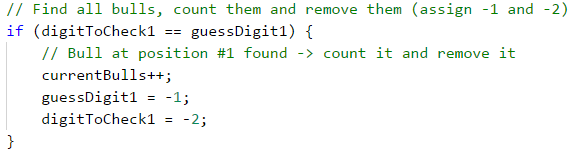We repeat the action for the second, third, and fourth digits.

Our conditional statement for cows can be done by this method - we check if the first digit from the generated number matches with the second, the third, or the fourth digit of the secret number. We can combine all conditional statements inside one condition, know that in the three cases we have a cow, but we won't know which digit to remove. That's why we type it one by one: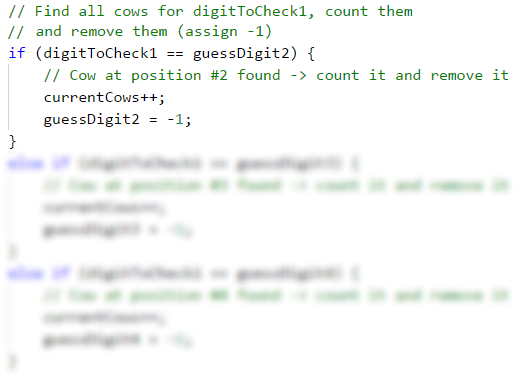After that, we sequentially check whether the second digit of the generated number matches the first one, the third one, or the fourth digit of the secret number; whether the third digit of the generated number matches the first one, the second one, or the fourth digit of the secret number; and finally, we check whether the fourth digit of the generated number matches the first one, the second one or the third digit of the secret number.

#### Printing The Output

After completing all conditions, we just need to check whether the bulls and cows in the currently generated number match the desired bulls and cows read from the console. If this is true, we print the current number on the console.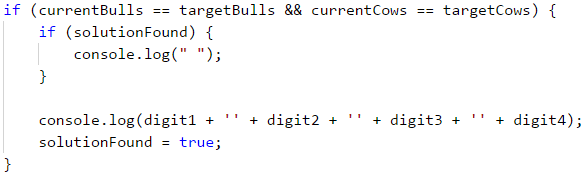### Testing in The Judge System

Test your solution here: https://judge.softuni.org/Contests/Practice/Index/942#2.